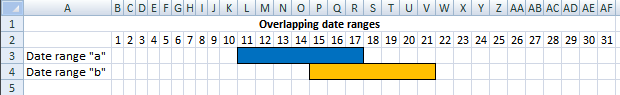Author: Oscar Cronquist Article last updated on January 11, 2019This blog article describes how to extract coinciding date ranges using array formulas, the image above shows random date ranges in cell range B3:C25.

Overlapping date ranges

Array formula in cell E4:

=SMALL(IF(COUNTIFS(\$B\$3:\$B\$25, "<="&\$C\$3:\$C\$25, \$C\$3:\$C\$25, ">="&\$B\$3:\$B\$25)>1, \$B\$3:\$B\$25, ""), ROW(A1))

To enter an array formula, type the formula in a cell then press and hold CTRL + SHIFT simultaneously, now press Enter once. Release all keys.

The formula bar now shows the formula with a beginning and ending curly bracket telling you that you entered the formula successfully. Don't enter the curly brackets yourself.

Copy cell E4 and paste down as far as needed.

Array formula in cell F4:

=SMALL(IF(COUNTIFS(\$B\$3:\$B\$25, "<="&\$C\$3:\$C\$25, \$C\$3:\$C\$25, ">="&\$B\$3:\$B\$25)>1, \$C\$3:\$C\$25, ""), ROW(A1))

Copy cell F4 and paste down as far as needed.

Date ranges without overlapping

Array formula in cell E17:

=SMALL(IF(COUNTIFS(\$B\$3:\$B\$25, "<="&\$C\$3:\$C\$25, \$C\$3:\$C\$25, ">="&\$B\$3:\$B\$25)=1, \$B\$3:\$B\$25, ""), ROW(A1))

Copy cell E17 and paste down as far as needed.

Array formula in cell F17:

=SMALL(IF(COUNTIFS(\$B\$3:\$B\$25, "<="&\$C\$3:\$C\$25, \$C\$3:\$C\$25, ">="&\$B\$3:\$B\$25)=1, \$B\$3:\$B\$25, ""), ROW(A1)) + CTRL + SHIFT + ENTER.

Copy cell F17 and paste down as far as needed.

### Explaining array formula in cell E4=SMALL(IF(COUNTIFS(\$B\$3:\$B\$25, "<="&\$C\$3:\$C\$25, \$C\$3:\$C\$25, ">="&\$B\$3:\$B\$25)>1, \$B\$3:\$B\$25, ""), ROW(A1))

Step 1 - Find overlapping date ranges

The COUNTIFS function calculates the number of cells across multiple ranges that equals all given conditions.

=SMALL(IF(COUNTIFS(\$B\$3:\$B\$25, "<="&\$C\$3:\$C\$25, \$C\$3:\$C\$25, ">="&\$B\$3:\$B\$25)>1, \$B\$3:\$B\$25, ""), ROW(A1))

COUNTIFS(\$B\$3:\$B\$25, "<="&\$C\$3:\$C\$25, \$C\$3:\$C\$25, ">="&\$B\$3:\$B\$25)

becomes

COUNTIFS({40109;40171;40210; 40264;40397;40405;40417;40457; 40500;40545;40611;40617;40726;40777;40791;40802;40831;40882;40994; 41014;41040;41127;41182}, "<="&{40137;40188;40268;40264;40401; 40413;40447;40517;40503; 40608;40613;40710;40773;40791;40817;40812;40875; 40927;41010;41085;41086;41168;41262}, \${40137;40188;40268;40264;40401; 40413;40447;40517;40503; 40608;40613;40710;40773;40791;40817;40812;40875;40927; 41010;41085;41086;41168;41262}, ">="&\${40109;40171;40210;40264;40397;40405; 40417;40457; 40500;40545;40611;40617;40726;40777;40791;40802;40831;40882;40994; 41014;41040;41127;41182}) and returns {1;1;2;2;1;1;1;2;2;1;1;1;1;2;3;2;1;1;1;2;2;1;1}

COUNTIFS(\$B\$3:\$B\$25, "<="&\$C\$3:\$C\$25, \$C\$3:\$C\$25, ">="&\$B\$3:\$B\$25)>1

returns

{FALSE;FALSE;TRUE; TRUE;FALSE;FALSE;FALSE;TRUE;TRUE;FALSE; FALSE;FALSE;FALSE;TRUE;TRUE;TRUE;FALSE;FALSE; FALSE;TRUE;TRUE;FALSE;FALSE}

Step 2 - Convert boolean array to start dates

The IF function has three arguments, the first one must be a logical expression. If the expression evaluates to TRUE then one thing happens (argument 2) and if FALSE another thing happens (argument 3).

=SMALL(IF(COUNTIFS(\$B\$3:\$B\$25, "<="&\$C\$3:\$C\$25, \$C\$3:\$C\$25, ">="&\$B\$3:\$B\$25)>1, \$B\$3:\$B\$25, ""), ROW(A1))

IF(COUNTIFS(\$B\$3:\$B\$25, "<="&\$C\$3:\$C\$25, \$C\$3:\$C\$25, ">="&\$B\$3:\$B\$25)>1, \$B\$3:\$B\$25, "")

becomes

IF({FALSE;FALSE;TRUE;TRUE; FALSE;FALSE;FALSE;TRUE;TRUE;FALSE; FALSE;FALSE;FALSE;TRUE;TRUE;TRUE;FALSE;FALSE; FALSE;TRUE;TRUE;FALSE;FALSE}, {40109;40171;40210; 40264;40397;40405;40417;40457;40500; 40545;40611;40617;40726;40777;40791;40802;40831;40882; 40994;41014;41040;41127;41182}, "")

and returns

{"";"";40210;40264; "";"";"";40457;40500;"";""; "";"";40777;40791;40802;"";"";""; 41014;41040;"";""}

Step 3 - Return the k-th smallest number in array

To be able to return a new value in a cell each I use the SMALL function to filter column numbers from smallest to largest.

The ROW function keeps track of the numbers based on a relative cell reference. It will change as the formula is copied to the cells below.

SMALL(array,k) returns the k-th smallest number in this data set.

=SMALL(IF(COUNTIFS(\$B\$3:\$B\$25, "<="&\$C\$3:\$C\$25, \$C\$3:\$C\$25, ">="&\$B\$3:\$B\$25)>1, \$B\$3:\$B\$25, ""), ROW(A1))

becomes

=SMALL({"";"";40210;40264;"";""; "";40457;40500;"";"";"";"";40777;40791;40802;"";"";"";41014; 41040;"";""}, ROW(A1))

becomes

=SMALL({"";"";40210;40264;"";""; "";40457;40500;"";"";"";"";40777;40791;40802;"";"";""; 41014;41040;"";""}, 1) and returns 40210 (1-Feb-2010)

### Get the Excel fileFilter-overlapping-date-ranges.xlsx